ML之LoR&Bagging&RF：依次利用Bagging、RF算法对泰坦尼克号数据集 (Kaggle经典案例)获救人员进行二分类预测——模型融合

## 输出结果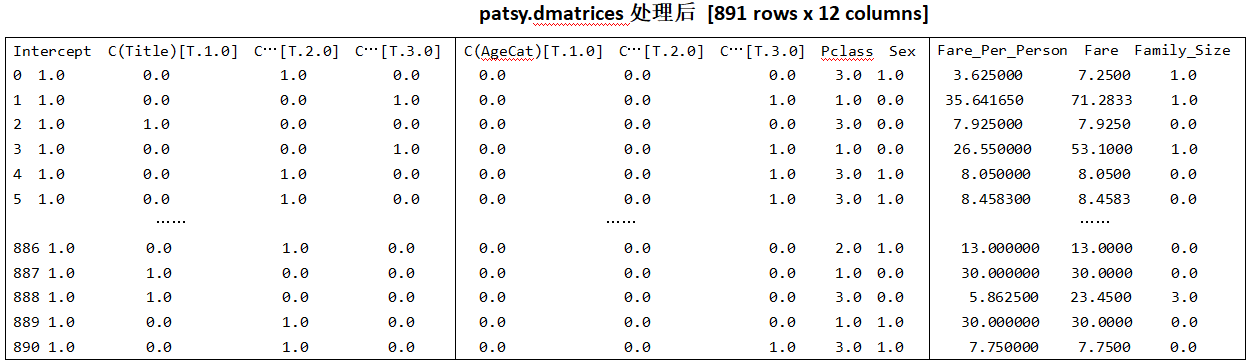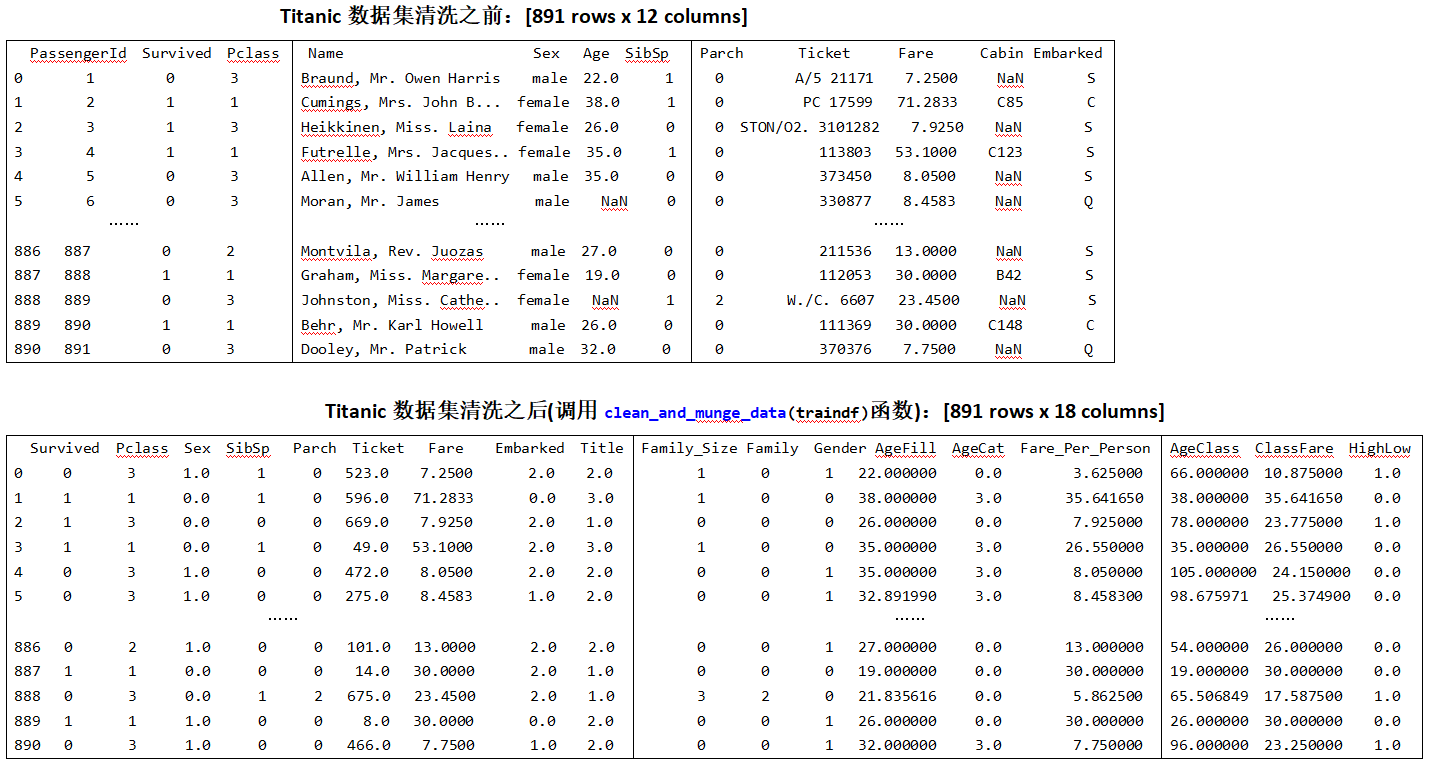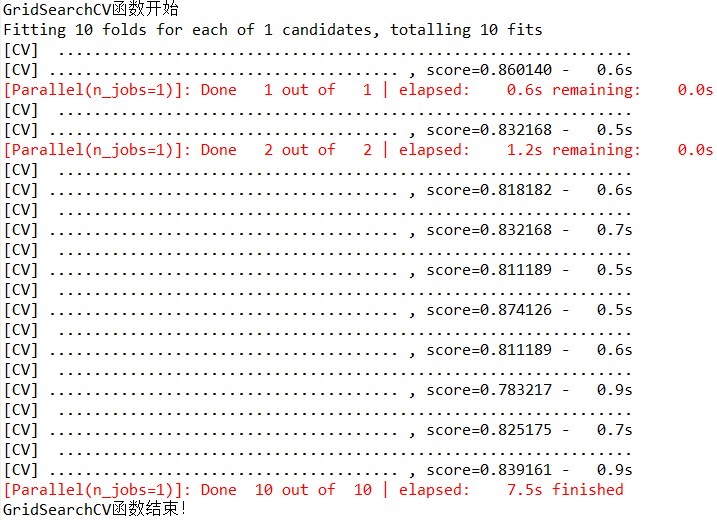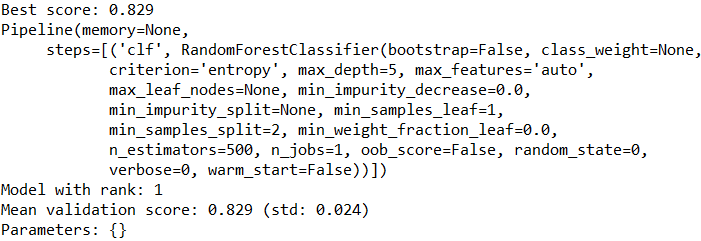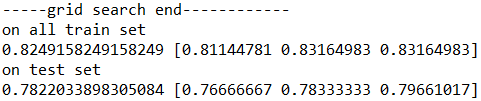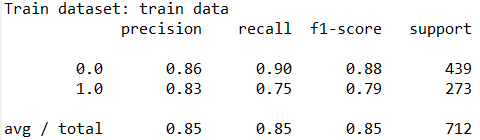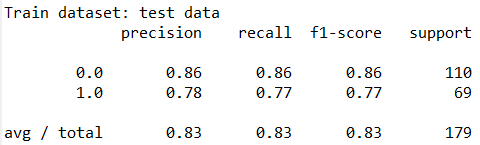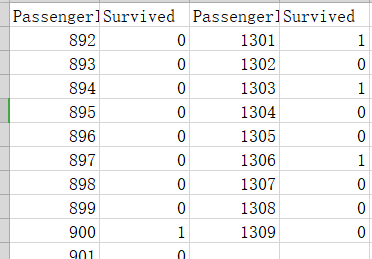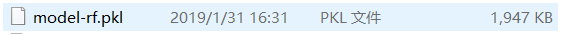## 设计思路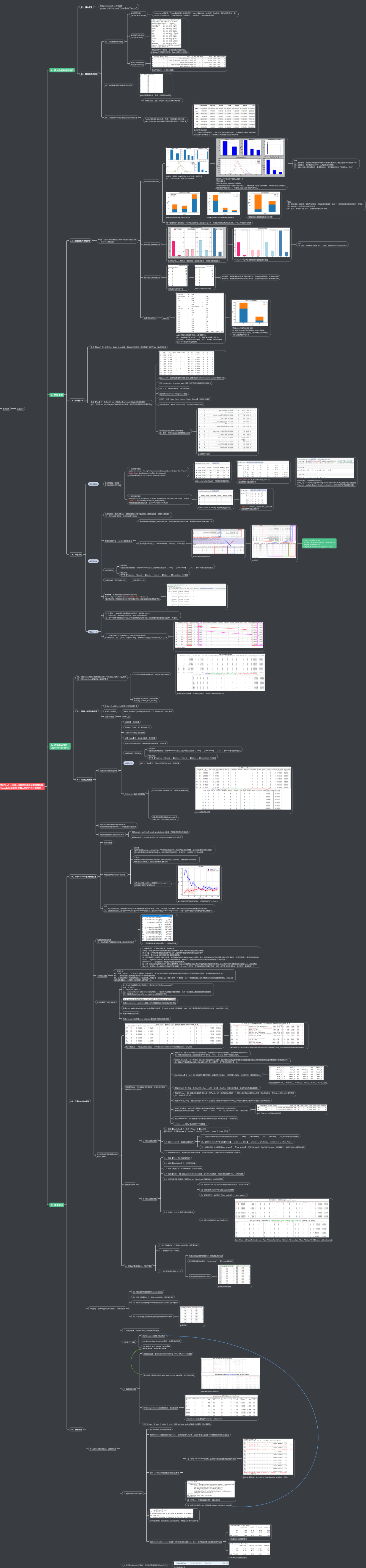## 核心代码

RF算法

```clf=RandomForestClassifier(n_estimators=500, criterion='entropy', max_depth=5, min_samples_split=2,
min_samples_leaf=1, max_features='auto',    bootstrap=False, oob_score=False, n_jobs=1, random_state=seed,
verbose=0)class RandomForestClassifier Found at: sklearn.ensemble.forestclass RandomForestClassifier(ForestClassifier):
"""A random forest classifier.

A random forest is a meta estimator that fits a number of decision tree
classifiers on various sub-samples of the dataset and use averaging to
improve the predictive accuracy and control over-fitting.
The sub-sample size is always the same as the original
input sample size but the samples are drawn with replacement if
`bootstrap=True` (default).

Read more in the :ref:`User Guide <forest>`.

Parameters
----------
n_estimators : integer, optional (default=10)
The number of trees in the forest.

criterion : string, optional (default="gini")
The function to measure the quality of a split. Supported criteria are
"gini" for the Gini impurity and "entropy" for the information gain.
Note: this parameter is tree-specific.

max_features : int, float, string or None, optional (default="auto")
The number of features to consider when looking for the best split:

- If int, then consider `max_features` features at each split.
- If float, then `max_features` is a percentage and
`int(max_features * n_features)` features are considered at each
split.
- If "auto", then `max_features=sqrt(n_features)`.
- If "sqrt", then `max_features=sqrt(n_features)` (same as "auto").
- If "log2", then `max_features=log2(n_features)`.
- If None, then `max_features=n_features`.

Note: the search for a split does not stop until at least one
valid partition of the node samples is found, even if it requires to
effectively inspect more than ``max_features`` features.

max_depth : integer or None, optional (default=None)
The maximum depth of the tree. If None, then nodes are expanded
until
all leaves are pure or until all leaves contain less than
min_samples_split samples.

min_samples_split : int, float, optional (default=2)
The minimum number of samples required to split an internal node:

- If int, then consider `min_samples_split` as the minimum number.
- If float, then `min_samples_split` is a percentage and
`ceil(min_samples_split * n_samples)` are the minimum
number of samples for each split.

.. versionchanged:: 0.18

min_samples_leaf : int, float, optional (default=1)
The minimum number of samples required to be at a leaf node:

- If int, then consider `min_samples_leaf` as the minimum number.
- If float, then `min_samples_leaf` is a percentage and
`ceil(min_samples_leaf * n_samples)` are the minimum
number of samples for each node.

.. versionchanged:: 0.18

min_weight_fraction_leaf : float, optional (default=0.)
The minimum weighted fraction of the sum total of weights (of all
the input samples) required to be at a leaf node. Samples have
equal weight when sample_weight is not provided.

max_leaf_nodes : int or None, optional (default=None)
Grow trees with ``max_leaf_nodes`` in best-first fashion.
Best nodes are defined as relative reduction in impurity.
If None then unlimited number of leaf nodes.

min_impurity_split : float,
Threshold for early stopping in tree growth. A node will split
if its impurity is above the threshold, otherwise it is a leaf.

.. deprecated:: 0.19
``min_impurity_split`` has been deprecated in favor of
``min_impurity_decrease`` in 0.19 and will be removed in 0.21.

min_impurity_decrease : float, optional (default=0.)
A node will be split if this split induces a decrease of the impurity
greater than or equal to this value.

The weighted impurity decrease equation is the following::

N_t / N * (impurity - N_t_R / N_t * right_impurity
- N_t_L / N_t * left_impurity)

where ``N`` is the total number of samples, ``N_t`` is the number of
samples at the current node, ``N_t_L`` is the number of samples in the
left child, and ``N_t_R`` is the number of samples in the right child.

``N``, ``N_t``, ``N_t_R`` and ``N_t_L`` all refer to the weighted sum,
if ``sample_weight`` is passed.

bootstrap : boolean, optional (default=True)
Whether bootstrap samples are used when building trees.

oob_score : bool (default=False)
Whether to use out-of-bag samples to estimate
the generalization accuracy.

n_jobs : integer, optional (default=1)
The number of jobs to run in parallel for both `fit` and `predict`.
If -1, then the number of jobs is set to the number of cores.

random_state : int, RandomState instance or None, optional
(default=None)
If int, random_state is the seed used by the random number
generator;
If RandomState instance, random_state is the random number
generator;
If None, the random number generator is the RandomState instance
used
by `np.random`.

verbose : int, optional (default=0)
Controls the verbosity of the tree building process.

warm_start : bool, optional (default=False)
When set to ``True``, reuse the solution of the previous call to fit
and add more estimators to the ensemble, otherwise, just fit a whole
new forest.

class_weight : dict, list of dicts, "balanced",
"balanced_subsample" or None, optional (default=None)
Weights associated with classes in the form ``{class_label: weight}``.
If not given, all classes are supposed to have weight one. For
multi-output problems, a list of dicts can be provided in the same
order as the columns of y.

Note that for multioutput (including multilabel) weights should be
defined for each class of every column in its own dict. For example,
for four-class multilabel classification weights should be
[{0: 1, 1: 1}, {0: 1, 1: 5}, {0: 1, 1: 1}, {0: 1, 1: 1}] instead of
[{1:1}, {2:5}, {3:1}, {4:1}].

The "balanced" mode uses the values of y to automatically adjust
weights inversely proportional to class frequencies in the input data
as ``n_samples / (n_classes * np.bincount(y))``

The "balanced_subsample" mode is the same as "balanced" except
that
weights are computed based on the bootstrap sample for every tree
grown.

For multi-output, the weights of each column of y will be multiplied.

Note that these weights will be multiplied with sample_weight
(passed
through the fit method) if sample_weight is specified.

Attributes
----------
estimators_ : list of DecisionTreeClassifier
The collection of fitted sub-estimators.

classes_ : array of shape = [n_classes] or a list of such arrays
The classes labels (single output problem), or a list of arrays of
class labels (multi-output problem).

n_classes_ : int or list
The number of classes (single output problem), or a list containing the
number of classes for each output (multi-output problem).

n_features_ : int
The number of features when ``fit`` is performed.

n_outputs_ : int
The number of outputs when ``fit`` is performed.

feature_importances_ : array of shape = [n_features]
The feature importances (the higher, the more important the feature).

oob_score_ : float
Score of the training dataset obtained using an out-of-bag estimate.

oob_decision_function_ : array of shape = [n_samples, n_classes]
Decision function computed with out-of-bag estimate on the training
set. If n_estimators is small it might be possible that a data point
was never left out during the bootstrap. In this case,
`oob_decision_function_` might contain NaN.

Examples
--------
>>> from sklearn.ensemble import RandomForestClassifier
>>> from sklearn.datasets import make_classification
>>>
>>> X, y = make_classification(n_samples=1000, n_features=4,
...                            n_informative=2, n_redundant=0,
...                            random_state=0, shuffle=False)
>>> clf = RandomForestClassifier(max_depth=2, random_state=0)
>>> clf.fit(X, y)
RandomForestClassifier(bootstrap=True, class_weight=None,
criterion='gini',
max_depth=2, max_features='auto', max_leaf_nodes=None,
min_impurity_decrease=0.0, min_impurity_split=None,
min_samples_leaf=1, min_samples_split=2,
min_weight_fraction_leaf=0.0, n_estimators=10, n_jobs=1,
oob_score=False, random_state=0, verbose=0, warm_start=False)
>>> print(clf.feature_importances_)
[ 0.17287856  0.80608704  0.01884792  0.00218648]
>>> print(clf.predict([[0, 0, 0, 0]]))


Notes
-----
The default values for the parameters controlling the size of the trees
(e.g. ``max_depth``, ``min_samples_leaf``, etc.) lead to fully grown and
unpruned trees which can potentially be very large on some data
sets. To
reduce memory consumption, the complexity and size of the trees
should be
controlled by setting those parameter values.

The features are always randomly permuted at each split. Therefore,
the best found split may vary, even with the same training data,
``max_features=n_features`` and ``bootstrap=False``, if the
improvement
of the criterion is identical for several splits enumerated during the
search of the best split. To obtain a deterministic behaviour during
fitting, ``random_state`` has to be fixed.

References
----------

..  L. Breiman, "Random Forests", Machine Learning, 45(1), 5-32,
2001.

See also
--------
DecisionTreeClassifier, ExtraTreesClassifier
"""
def __init__(self,
n_estimators=10,
criterion="gini",
max_depth=None,
min_samples_split=2,
min_samples_leaf=1,
min_weight_fraction_leaf=0.,
max_features="auto",
max_leaf_nodes=None,
min_impurity_decrease=0.,
min_impurity_split=None,
bootstrap=True,
oob_score=False,
n_jobs=1,
random_state=None,
verbose=0,
warm_start=False,
class_weight=None):
super(RandomForestClassifier, self).__init__
(base_estimator=DecisionTreeClassifier(), n_estimators=n_estimators,
estimator_params=("criterion", "max_depth", "min_samples_split",
"min_samples_leaf", "min_weight_fraction_leaf", "max_features",
"max_leaf_nodes", "min_impurity_decrease", "min_impurity_split",
"random_state"), bootstrap=bootstrap, oob_score=oob_score,
n_jobs=n_jobs, random_state=random_state, verbose=verbose,
warm_start=warm_start, class_weight=class_weight)
self.criterion = criterion
self.max_depth = max_depth
self.min_samples_split = min_samples_split
self.min_samples_leaf = min_samples_leaf
self.min_weight_fraction_leaf = min_weight_fraction_leaf
self.max_features = max_features
self.max_leaf_nodes = max_leaf_nodes
self.min_impurity_decrease = min_impurity_decrease
self.min_impurity_split = min_impurity_split```